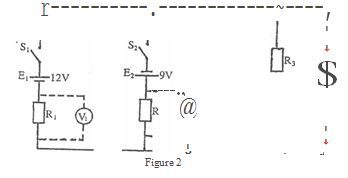Applied Electricity 1 , May/June 2010
 Questions: 1 2 Main
General Comments
Weakness/Remedies
Strength

Question 2(a) Connect the circuit as shown in Fig.2.
(b) Ask the Supervisor to check the circuit.
(c) Copy Table 2 .

Table 3

 Vl(v) V2(v) V3(v) Voltmeter reading

(d) Close the switches Sl and S2'
(e) Measure, read and record in Table 2 the voltage drop across Rl.
(f) Repeat step (e) for R2 and R3•
(g) Evaluate El + VI'
(h) Evaluate E2 + V2.
(i) Compare the values obtained in steps (g) and (h) with V3.
(j) What is your conclusion?
(k) Evaluate:
(i) El + E2;
(ii) VI + V2•
(I) Comment on your results in (k).
(m) Which law does your result in (k) obey?

_____________________________________________________________________________________________________
Observation

The candidates were required to
(i) connect the circuit as shown by the way of changing the terminals of the voltage
sources;
(ii)           evaluate the voltage drops across each branch in the circuit;
(iii)        evaluate like parallel voltages in the circuit;;
(iv)        comment on and state the law that the like parallel voltages obey.
The expected answers were:

 Vl(v) V2(v) V3(v) Voltmeter reading -13.714 7.286 -1.714

(k) (i) El + E2 = (12 + 9) V = 21 V
(ii)Vl + V2 = 6.428 V
(I) The e.m.f. sum and the voltages sum are not the same

(m) It obeys Kirchhoff's Voltage Law.
The candidates understood the task, connected the circuit and obtained readings. Again, an inaccurate reading was the undoing of those whose performances were low. Another noticed weakness in question 2 is the inability to state accurately the law that governs the experiment.

 Powered by Sidmach Technologies(Nigeria) Limited . Copyright © 2015 The West African Examinations Council. All rights reserved.